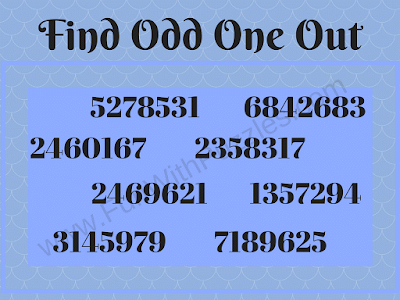Recently we have posted Odd One Out Puzzles. Many of these Odd One Out Puzzles are of Visual Puzzle types which some pictures are given and one has to find out the odd picture among these pictures which will be slightly different from the other pictures. Recently we have also posted a few Odd One Out Number puzzles in which one has to find the Odd Number out among the given set of numbers. These puzzles were from easy difficulty level to medium level. Today we are posting the Odd One Puzzles which are of high difficulty level. Hence we have marked these puzzles for adults as kids and teens will find these puzzles very difficult to solve.
In these Odd One Out Puzzles, eight numbers are provided in each of the puzzles. Seven number out of these eight numbers have something in common. However, there is only one number that does not follow the required rules or property which the other seven numbers follow. One has to first find this similarity among these numbers and thus mark the odd number out.
We will be posting the answers to these puzzles soon. Meanwhile, try writing your answers in the comment section of this post.1. Odd One Out Picture Puzzle2. Odd One Out Tough Picture Brain Teaser3. Odd One Out Tough Picture Puzzle4. Odd One Out Tough Picture Riddle5. Odd One Out Tough Picture Question

Anonymous said...